# Gate Mock Test- 13: CS/IT

## 65 Questions MCQ Test GATE Computer Science Engineering(CSE) 2023 Mock Test Series | Gate Mock Test- 13: CS/IT

Description
Attempt Gate Mock Test- 13: CS/IT | 65 questions in 180 minutes | Mock test for GATE preparation | Free important questions MCQ to study GATE Computer Science Engineering(CSE) 2023 Mock Test Series for GATE Exam | Download free PDF with solutions
QUESTION: 1

### Direction: Two delivery boys Ram and Amar use their scooters to deliver pizza to different places in city Delhi. The line graph given below represents the distance travelled by them in months from January to June.With the help of given data answer the following questions: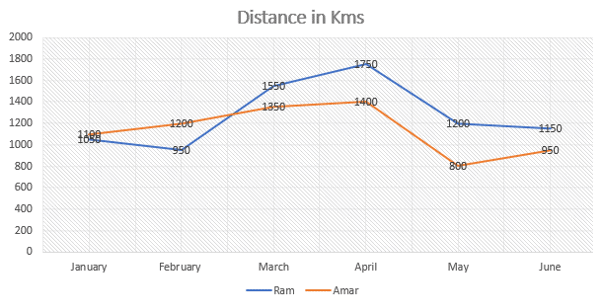The distance travelled by Ram in the months of Feb and May is what percent less/more than the distance travelled by Amar in Feb and May?

Solution:

The distance travelled by Ram in February = 950 km

Distance travelled by him in month of May = 1200

Total distance travelled by Ram in Feb and May = 950+1200 = 2150 kms

The distance travelled by Amar in February = 1200 km

Distance travelled by him in month of May = 800

Total distance travelled by Amar in Feb and May = 1200+800 = 2000 kms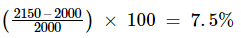The distance travelled by Ram in Feb and May is 7.5% more than distance travelled by Amar in Feb and May.

Hence, option (c) is correct.

QUESTION: 2

### Which of the following refers to ‘To fall asleep from exhaustion?

Solution:

‘Flake out’ means to fall asleep from exhaustion.

Fall back on - begin to use something held in reserve

Fritter away - To waste or squander time or money.

Ferret out - To discover something after searching.

QUESTION: 3

### Direction: Study the information carefully to answer the questions that follow.In Vimla public school, students can choose among three sports Cricket, Football and Hockey. Out of 1000 students, each one is required to play at least one of the three sports. 40 students play all three sports. 380 students play only Hockey, 170 students play only Cricket, 60 students play exactly two sports Hockey and cricket and 240 students play only Football.What is the number of students who play exactly two sports?

Solution:

It is given that the total number of students are = 1000

Only Hockey = 380

Only Cricket = 170

Only Football = 240

Hockey + Cricket + Football = 40 {all the three sports}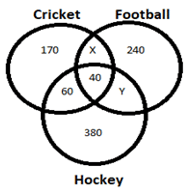Now, let X be the number of students who play Cricket and Football.

And Y is the number of students who play football and hockey.

Now total number of students,

170 +60 +40 +380+ 240+ X + Y= 1000

X + Y = 110

And 60 students play only hockey and cricket, and that number we get after removing students who play all three sports from students who play hockey and cricket.

X + Y + 60 = 110+ 60 = 170

There are 170 students who play exactly two sports.

QUESTION: 4

He lost the game _________ he has improved a lot

Solution: Nonetheless can go at the end of a sentence. This sentence can also be written as: He lost the game; nonetheless, he has improved a lot.
QUESTION: 5

Rs. 200 are divided among Arun, Bholu and Chetan such that Arun's share is Rs. 30 more than Bholu and Rs. 20 less than Chetan's. What is Bholu's share?

Solution: It is given that Rs. 200 divided among Arun, Bholu and Chetan

Let Bholu’s share = X Rs.

Arun’s Share = X + 30 Rs

Chetan’s Share = X + 30 + 20 = X+50

Now, Arun+ Bholu+ Chetan = (X + 30) + (X) + (X + 50) = 200

3X = 200 – 80

X = 120/3

X = 40

Bholu’s share is 40 Rs.

Hence, (b) is the correct option.

QUESTION: 6

The average of 5 consecutive integers starting with 'm' is n. What is the average of 6 consecutive integers starting with (m+2)?

Solution:

According to the question let m = 1

∴ 5 consecutive integers are = 1, 2, 3, 4, 5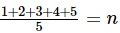n = 15/5 = 3

6 consecutive integers starting with (m+2) are = 3, 4, 5, 6, 7, 8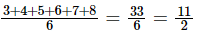Now check from option to put n = 3

Option : (a)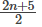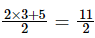(Satisfied)

Hence, the correct option is (a).

QUESTION: 7

Ramesh sold a television for Rs 18500 at a loss of 7.5%. If he wants to earn 16% profit by selling the television then at what price he should sell the television?

Solution:

Cost price of the television =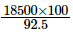= Rs. 20000

To earn 16% profit

Selling price =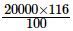= Rs. 23200

QUESTION: 8

A sum of money at compound rate of interest amounts to Rs. 750 in 1st year and to Rs. 1000 in 2nd year. Find the sum:

Solution:

Amount after 1st year serves as principal for 2nd year. So,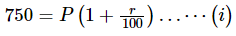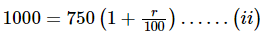Dividing (?) by (??) we get,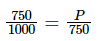P=Rs.562.50

QUESTION: 9

Present average age of Kanika and Shweta is ‘x’ years. Ratio of the age of Kanika one year ago to the age of Monika four year hence will be 1: 2. Present average age of Kanika, Monika and Shweta is 22 years. Find the value of ‘x’ if the present age of Kanika is 16 years

Solution:

Present age of Kanika = 16 years

Age of Kanika one year ago =16-1=15 years

Age of Monika after four years = 15×2=30 years

Present age of Monika = 30-4=26 years=26 years

e present ages of Kanika, Monika and Shweta =22×3=66 years

Present age of Shweta =66-16-26-24 years

Present average age of Kanika and Shweta =x=(24 + 16)/2=20 years

So, the value of ‘x’ is 20 years

So option (d) is the correct answer.

QUESTION: 10

The HCF and LCM of two positive numbers are 5 and 585. If two numbers are in the ratio 9: 13, then find the sum of numbers.

Solution:

Let the two numbers be 9X and 13X

So, (9x) × (13x)= 5 × 585

117x2 = 25 x 117

x2=25

X = 5

Required sum =9 × 5 + 13 × 5 = 45 + 65 = 110

So option (a) is the correct answer.

QUESTION: 11

From the types of sort given below choose the sort from which we get best average behavior

Solution: We can expect the best average behavior from Quick sort because it uses the concept of partitioning . Hence option b is correct
QUESTION: 12

Consider an array consisting of the following elements in unsorted order (placed randomly), but 60 as the first element.

60, 80, 15, 95, 7, 12, 35, 90, 55

Quicksort partition algorithm is applied by choosing the first element as the pivot element. How many total number of arrangements of array integers is possible preserving the effect of first pass of partition algorithm.

Solution: We have to choose the first element as pivot.

Here 60 is given as the first element.

After the first pass, the pivot element goes to it’s exact location.

Here 60 goes to 6th place.

All the elements less than 60 go to the left of 60 and all the elements greater than 60 go to the right of 60.

After 1st pass

⇒ 5! × 3!

⇒720 possible arrangements.

QUESTION: 13

The grammar which is equivalent to -

E → E+/ES+/+

S → */S*

After eliminating the left recursion is-

E' → S+E'/+E'

E' → EPSILON

S → *S'

S' → *S'/EPSILON

E' → S+E'/+E'

S → *S'

S' → *S'/EPSILON

E' → +E'/EPSILON

S → *S'

S' → *S'/EPSILON

Solution:

The way of removing left recursion is-

A→ AB / C is replaced by A → CA'

A' → BA' / epsilon

So the answer is - E → +E'

E' → S+E'/+E'

E' → EPSILON

S → *S'

S' → *S'/EPSILON

QUESTION: 14

Consider the code segment

int i, j, x, y, m, n;

n = 20;

for (i = 0, i < n; i++)

{

for (j = 0; j < n; j++)

{

if (i % 2)

{

x + = ((4*j) + 5*i);

}

}

}

m = x + y;

Which one of the following is false?

Solution:

int i,j,x,y,m,n;

N = 20;

for(i = 0; i < n; i++)

{

for(j = 0;j < n; j++)

{

if(i%2)

{

x + = 4*j[4<

y + = (7+4*j);

}

}

}

M = x + y;

QUESTION: 15

One piece of data is being sent from one host to another host through the HyperText Transfer protocol. What port number will be attached to destination port number?

Solution: HTTP uses port number 80 by default.
QUESTION: 16

A bit-stuffing based framing protocol uses an 8-bit delimiter pattern of 01111110. If the output bit-string after stuffing is 01111100101, then the input bit-string is

Solution:

Given 8 – bit delimiter pattern of 01111110.

Output Bit string after stuffing is 01111100101

StuffedBit

Now, Input String is 0111110101.

QUESTION: 17

Consider an Ethernet network employing CSMA/CD, where Bw = 10 Mbps, length of the cable (L) is 200m and v = 2 x 108 m/sec. Calculate the maximum frame size in bytes.

Solution: In CSMA/CD, Transmission time (Tt) = 2 × Tp

Therefore, maximum frame size = 2 × L × Bw / v

= 20 bits = 2.5 bytes.

QUESTION: 18

A system contains three programs and each requires three tape units for its operation. The minimum number of tape units which the system must have such that deadlocks never arise is a _________.

Solution: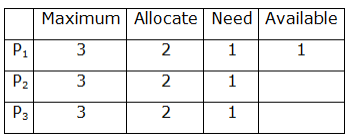With the above-given data, after allocating 2 units of tape to each process, with 1 available unit any of the 3 processes can be satisfied in such a way that no deadlock will be there. So the answer is 7 tape units.

QUESTION: 19

If F= {A →BC, CD → E, E →C, D → AEH, ABH →BD, DH → BC} then canonical cover over F is

Solution:

F= {A →BC, CD → E, E →C, D → AEH, ABH →BD, DH → BC} then a nonredundant cover for F { A → BC, E →C, D → AEH, ABH →BD }. Then FD ABH → BD can be decomposed into FDs ABH → B and ABH →D

Now since, the FD A →B and AH → D. We also notice that AH →B is redundant since the FD A → B is already in F

That gives us the canonical cover as {A → B, A→C, E → C, D →A, D → E, D →H, AH → D}.

QUESTION: 20

One to many relationship between X(one side) and Y(many side) entities in an E-R diagram is converted as

Solution: The E-R model can be represented with two relations X and Y therefore Y side includes the primary key of X side as foreign key.
QUESTION: 21

In ER models, which statement is not valid?

Solution: It is seen that in ER model, entity is present in ER model while relational table is available in Relational Model. From above options we see that option (A) and option (B) are true as ER model supports multi valve and composite attributes, with this we see that option (C) is false. In case of option (D) we see that this option is true as an entry in the relational table has exactly one NULL value.
QUESTION: 22

Consider the following SQL query

SELECT studentid, studentname

FROM student

WHERE birthyear <=ALL (SELECT birth year from student)

Identify the answer returned by the above query?

Solution: Given SQL query prints the oldest student information.
QUESTION: 23

A person is having 10 flowers in which 3 are of the same type A and 2 are of the same type B. He wants to make a garland out of all the flowers he is having. How many types of Garland are possible?

Solution: 3 are of type A and 2 are of type B so the possible garlands are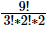= 15120
QUESTION: 24

From the letters of the word ‘INSTITUTION’, let the number of words in which all I’s come together possible from the permutation of the letters is X and the number of words in which vowels come at even position possible from the permutation of the letters is Y, then what is X - Y?

Solution: If all I's should be together then we make it a single unit '(III) TTTNNSUO' so now we've to arrange 9 units in which there are 3 T's and 2N 's so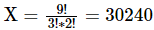. There are 11 places in which position 2,4,6,8,10 are even and we need to place a vowel at an even position.

Therefore X - Y = 29040

QUESTION: 25

If we run Dijkstra algorithm on vertex S to the following graph, then which of the following is shortest path distance from S to t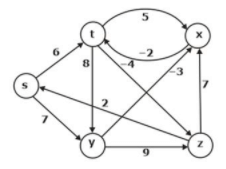Solution: When we run the dijkstra algorithm on vertex S, it will explore all edges which are reachable from S and then we will choose an edge which has minimum weight. So < s, t > edge having minimum weight is 6.
QUESTION: 26

Consider the following elements:

5 , 12 , 3 , 15 , 4 , 6 , 10

These elements are inserted one-by-one into two separate heaps, one min-heap and another max heap. Then these two heaps are stored in two different arrays starting from index How many elements have the same index value in both the arrays _______.

Solution: Min-Heap after all insertions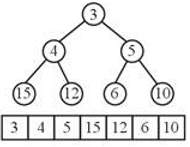Max-Heap after all insertions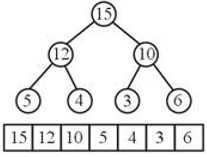Clearly, no element has the same index.

QUESTION: 27

Consider a relation R related to 7 entities E1,E2…..E7 respectively, each of them has 2 attributes (A,B) (C,D) (E,F) (G,H) (I,J) (K,L) (M,N) with first attribute as candidate key in all entities. All entities are related to R with one-one mapping. What is the number of candidate keys possible for R :

Solution: For one to one mapping b/w a relation & entity set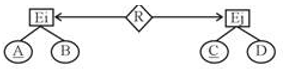No. of candidate keys at R = {A.C} i.e. 2

Therefore for a relation related to 7 entities

No. of candidate keys = 7 {A, C, E, G, I, K, M}

QUESTION: 28

Consider a case in which A and B are been considered as non zero +ve integers, then find out the outcome of following Pascal program

While A < > B do

if A < B then

A : = A – B

else

B : = B – A;

Write(A);

Solution:

We need to apply Optional Elimination

Taking A = 6 AND B = 4

Case it’s GCD when GCD = 2

Now dry run the code

1 → A = 2, B = 4

2→ A = 2, B = 2

Prints A which is 2 which means it’s a GCD

QUESTION: 29

Consider the following elements in a heap {17, 9, 6, 3, 12, 2, 15, 14, 20, 13, 21}. The minimum number of exchanges to convert it into a min heap be P. Then the minimum number of elements that can be arranged in a BST of height P is

Solution: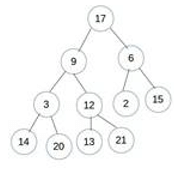From the given heap, it is obvious that 3 and 9 need to be exchanged for 17 and 3, but there are also exchanges needed for 2 and 6. So, 2 should be at the root as it is the minimum. Since the minimum number of exchanges is asked, it is clear that if exchanges are carried out in this manner, 2 – 6, 2 – 17, 6 – 17 and 3 – 9 separately, then the number of exchanges required = 4. But in other way, exchanges would be like:

3 – 9, 3 – 17, 9 – 17, (3 is at root now) and on the right side:

2 – 6, 2 – 3. Thus 5 exchanges. So P = 4.

But we have to find the minimum number of exchanges.

Now the elements in BST with height 4 is 4+1(root) = 5

QUESTION: 30

Consider the following statement:

S1: Deletion in referencing relation causes deletion anomaly.

S2: Referential integrity constraints are specified between exactly two relations in a schema.

S3: Count(*) also counts the tuples which consist of NULL in a relation.

S4: Every relation is possible to decompose into BCNF with dependency preserving and lossless join decomposition.

Which option is correct?

Solution:

S1: Referencing relation is the relation referring to a primary key of some other relation. Deletion in that table will not cause any violation. Hence, no deletion anomaly hence this is true.

So, this statement is true.

S2: This statement is true, since foreign key can be created within a relation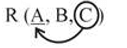C is a foreign key referring to the primary key "A'' of the same relation R.

S3: S3 is true since count (*) will count the no. of tuples in a relation, on the basis of ID value.

S4: It is true. Since it is possible to decompose every relation into BCNF with lossless join decomposition property but it may or may not preserve the dependencies of the relation.

QUESTION: 31

Given a 2D integer array as arr[0-99][0-99] , with base address of 900. A user decides to fill data from arr instead of arr. So he fills entries arr, arr , arr , ………….. , arr , then again from arr , arr , arr and so on. Given that size of integer is 4 Bytes and row major ordering is used. What is the address of 121st entry ? (entries counted as 1 , 2 , 3 and so on)

Solution:

1 entry arr

2 entry arr

3 entry arr

.

.

.

100 entry arr

101 entry arr

102 entry arr

.

.

.

121 entry arr

Hence 121st entry is at arr

As we know in a 2D array as arr[0-i][0-k]

arr[m][n] = base address + [m*(k+1) + n]*size of element

Hence ,arr = 900 + [(16*100) + 20]*4

= 7380

QUESTION: 32

Consider the following Turing Machine.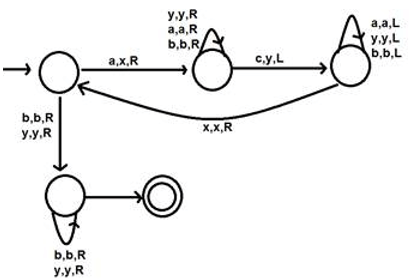A Turing machine either halts or hangs on a specific input. When a Turing machine hangs, then the input string is rejected because the further processing of string cannot be done. When a Turing machine halts, it may halt by accepting the input or may halt by rejecting the input. If it halts in the final state, then the input is accepted, otherwise rejected. On which input, the above Turing machine will halt in a final state and accept that string?

Solution: It is observed that all the b's are skipped while comparing a & c of the given input string. Hence this turning machine checks for the equivalence of a & c & skip b's.

∴ language accepted by turing machine = {anbmcn}

QUESTION: 33

An undirected graph consists of 50 vertices and 150 edges. Weight of the MST of this graph is 300. Each weight of each edge of this graph is incremented by 5, now what is the updated weight of the MST?

Solution: As the number of vertices is 50 so, the number of edges included in our MST would be 49 and each edge is incremented by 5. Total weight incremented = 49 5 = 245.

On adding the previous weight of the MST i.e. 300 to it we get 545 the answer!

QUESTION: 34

Load the keys 23, 13, 21, 14, 7, 8, and 15 in this order, in a hash table of size 7 using quadratic probing with c(i)= ±i^2 the hash function h(key) = key % 7.

[Note: The required probe sequences are given by: h_i (key)= (h(key)±i^2 )%7,i=0,1,2,3,....]

How many collisions can occur in the hash table?

Solution: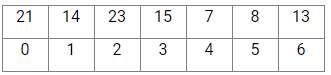23 ⇒ No collision

13 ⇒ No collision

21 ⇒ No collision

14 ⇒ 1 collision

7 ⇒ 2 collisions

7%7 = 1 (Collision)

[(7%7) + 1] mod 7 = 1 (Collision)

[(7%7) + 4] mod 7 = 4

Similarly, 8 ⇒ 2 collisions

15 ⇒ 3 collisions.

Therefore, total collisions are: 1 + 2 + 2 + 3= 8

QUESTION: 35

Match List-I (Dynamic algorithm) with List-II (Average case running time) and select the correct answer using the codes given below the lists: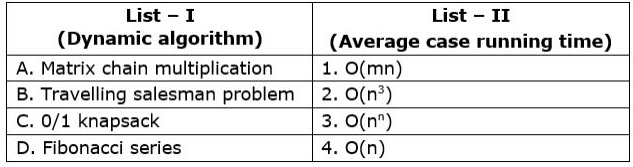Solution:

A) Matrix chain multiplication : (n3)

B) Travelling salesman problem : (nn)

C) 0/1 knapsack : (mn)

D) Fibonacci series : Ο(n)

QUESTION: 36

Determine the width of Micro-instruction having following Control signal field, in a vertical Micro-programmed Control Unit

a) Next Address field of 5 Bits

b) ALU Function field selecting 1 out of 7 ALU Function

c) Register-in field selecting 1 out of 15 Registers

d) Register-out field selecting 1 out of 15 Registers.

e) Shifter field selecting no shift, right shift or left shift

f) Auxiliary control field of 3 bits

Solution:

Next Address field = 5 bits

ALU Function field is of 3 bits (to select 1 out of 7 ALU Functions)

Register-in and Register-out Fields of 4 bits each

Shifter Field of 2 bits to select 1 out of three possibilities

Auxiliary Field of 3 bits

Total bits in microinstruction = 5 + 3 + 4 + 4 + 2 + 3 = 21.

QUESTION: 37

Which of the following is true regarding the following schedule S with 4 transactions?

S: r1(a); r2(b); w2(a); w2(b); w3(a); r3(c); w3(b); r1(c); w4(b); w3(c); r4(a); r4(c); w4(c); commit1; commit2;

commit3; commit4;

Solution:

The precedence graph for the schedule S is given as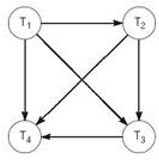There is no cycle in the precedence graph so it is conflict serializable. All conflict serializable schedules are also viewed as serializable.

From the graph, it is clear that is possible but is not possible.

QUESTION: 38

The micro instructions stored in the control memory of a process or have a width of 26 bits. Each micro instruction is divided into three fields: a micro-operation field of 13 bits, a next address field (X), and a MUX select field (Y). There are 8 status bits in the inputs of the MUX.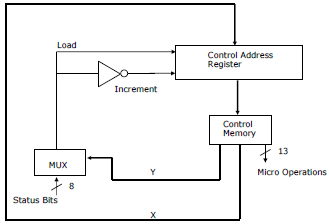How many bits are there in the X and Y fields, and what is the size of the control memory in the number of words?

Solution:

Control memory processor has 26 bit microinstruction divided into three field microoperation field is 13.

So, next address field (X) = 10

MAX select field = 3

Size of control = 210 = 1024

QUESTION: 39

Consider a hash table of size 10 in which linear probing is used to avoid collisions. The hash function used is HF(key) %10. Now consider the following hash table after insertion of these keys: 42,23,34,52,46,33 not necessarily in the same order.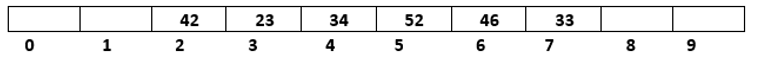Find the number of input sequences possible that results in the same hash table as above.

Solution:

Here keys are given in the sequence

42, 23, 34, 52, 46, 33

to get the output as above has a table, 42 must occur before 52 since the hash table is filled using linear probing.

Also, (42, 23, 34) should come before 52, but these 3 values can come in any order.

So, total possibilities = 3! = 6

Now 33 should occur at last of all the values. So, 33 is fixed to appear at 6th place.

Now 46 can appear at any of the remaining 5 positions.

Total possibility = 3! × 5 = 30

Hence, the answer would be 30.

QUESTION: 40

Which of the following statements about parser is/are CORRECT?

I. Canonical LR is more powerful than SLR

II. SLR is more powerful than LALR

III. SLR is more powerful than Canonical LR

Solution:

Bottom up parsers in decreasing order of their power: CLR≫ LALR≫ SLR≫ LR (0)

The given statements:

I. Canonical LR is more powerful than SLR is CORRECT.

II. SLR is more powerful than LALR is INCORRECT

III. SLR is more powerful than Canonical LR is INCORRECT.

QUESTION: 41

Consider the circuit shown in the figure below: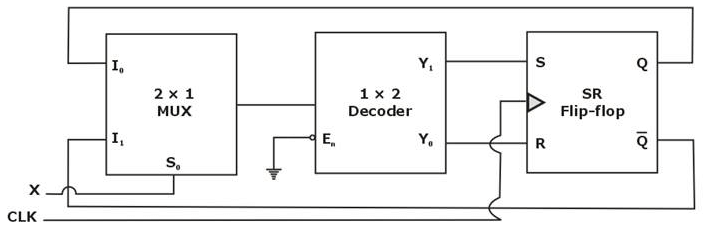The circuit can work as

Solution:

The 2 × 1 MUX m the circuit is working as art Ex-OR gate and 1 × 2 decoder as NOT gate,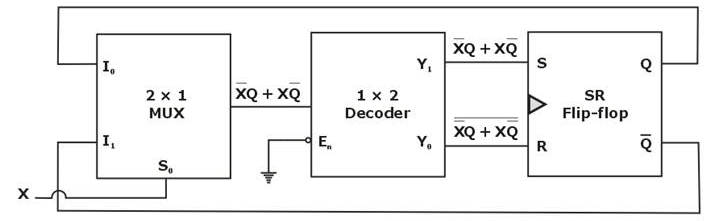Now the resulting figure can be drawn as shown below: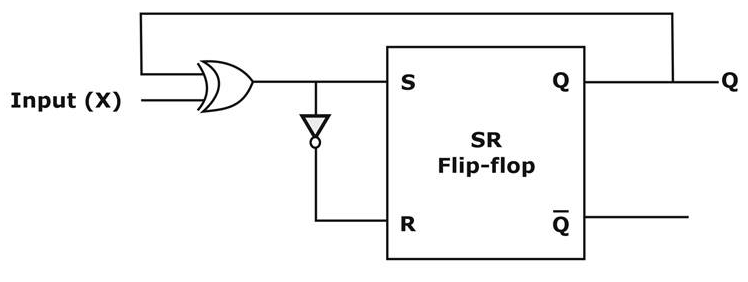S = X ⊕ Qn

R =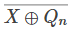For SR flip-flop,

Qn+1 = S +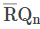= (X ⊕ Qn) +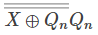= (X ⊕ Qn)(1 + Qn)

Qn+1 = X ⊕ Qn

Qn+1 is the excitation equation for T flip-flop. Thus, the circuit will function as a T flip-flop.

QUESTION: 42

The number of substrings (both trivial and nontrivial included) for a string containing n distinct characters is

Solution:

This is how we derive the number of substrings in a string of n characters. Substrings containing 0 letters = 1(ϵ)

1 letters = n

2 letters = n − 1

3 letters = n − 2

(n − 1) letters = 2

n letters =1

Add then up, 1 + (n + m − 1 + n − 2 +…….+ 3 + 2 + 1)

=1 + [n(n + 1)2]

Hence (d) is the most appropriate choice.

QUESTION: 43

Consider the following statements regarding the degree of the vertex in graph G.

S1: The number of odd degree vertices is always even in every graph G.

S2: In every simple undirected graph, at least 2 vertices must have the same degree.

S3: Every bipartite graph is bichromatic.

The number of true statements are _______.

Solution:

S1 is quite popular and easy to understand.

S2 however, is actually a corollary of the Havel-Hakimi Theorem, which says, there exists no graphical sequence with all distinct degrees.

S3 is also true, as every bipartite graph can be coloured using exactly 2 colours.

QUESTION: 44

Assume the base register contains 32856. The program counter is currently at 25687 memory location. What is the branch address if the address field of the jump instruction contains –30 in the address field and the instruction is designed with the base register addressing modes?

Solution: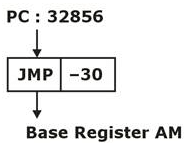Effective Address [EA] = [Base register] + Relative value

= 32856 + (-30) = 32826

PC = 32826

QUESTION: 45

Consider a system which has 28 instances of a resource P such that 4 + n processes share them, 4 processes request 5 instances of ‘P’. If n processes request 5 instances of same resources what is the maximum value of n such that system is in safe state ________

Solution:

28 > 4 × (5 × 1) + (5 – 1) × n

28 > 20 – 4 + 5n – n

28 > 16 + 4n

12 > 4n

3 > n

Maximum value of n is 3 – 1 = 2

QUESTION: 46

A user has been assigned an IP address 110.10.32.68/27. Which of the following IP addresses can be assigned for directed broadcasting in the same network.

Solution:

IP : 110.10.32.68

Net ID : 110.10.32.64

We have 5 bits for host ID. When all bits of host Id is 1 then it is a direct broadcasting address.

So,

\begin{tabular}{cc}

110.10 .32 .010 & 11111 \

\hline Network & Host \

bit & bit \

=110.10.32.95 &

\end{tabular}

So, option (c) is correct.

QUESTION: 47

Consider the following statements given below:

S1: There is always a lossless, dependency preserving BCNF decomposition.

S2: Any relation with two attributes is in BCNF.

S3: For two relations R(A, B) and functional dependency F = {A → B} and S(B, C) and functional dependency F = {B → C}, natural join is in BCNF.

Which of the following statements are true?

Solution:

S1 : Lossless dependency preserving BCNF decomposition is not always possible. S1 false

S2 : With two attributes a relation is always in. BCNF. S2 true

R(A, B)

A → B, B → A

Both A and B are key for R.

S3 : The natural join of R and S will have functional dependencies A → B and B → C, which is not in BCNF. S3 false

So option (D) is correct.

QUESTION: 48

Consider the following grammar:

S → Aa | bAc | dc | bda

A → e

Which of the following is correct about the above grammar?

Solution: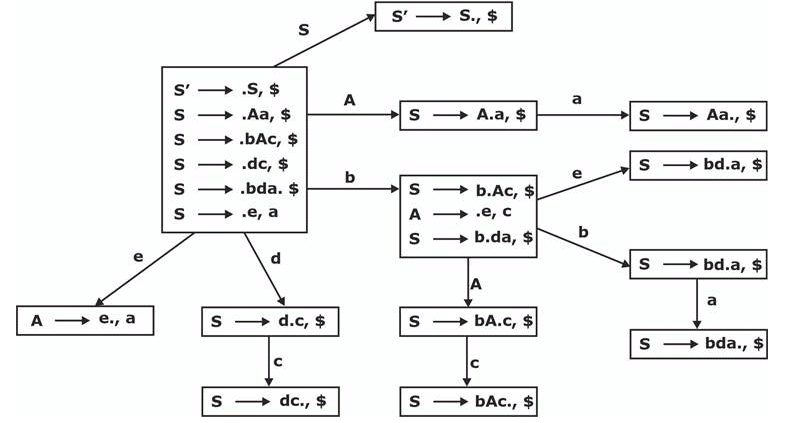There is no conflict in any of the state so it SLR, LALR(1), CLR(1).

For LL(1)

First(S) = First (AA) ∩ First (bAC) ∩ First (dC) ∩ First (bda)

= {e} ∩ {b} ∩ {d} ∩ {b} ∩ Φ

Not LL(1).

QUESTION: 49

Out of all possible three digit numbers, a number is picked at random. The probability that the number does not contain the digit 6 is _______.

Solution:

Total number of 3 digit numbers = 900 (Digits from 100 to 999)

At first place, 0 and 6 aren't allowed so 8 choices for filling first place; for second and third place, all digits are allowed except the digit 6, hence 9 choices for filling second and third place.

n(Not containing the digit 6) = (8 x 9 x 9)

P( Not containing the digit 6) =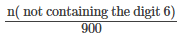=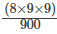Solve this to get 0.72 as the answer.

QUESTION: 50

Consider the following function, to which a pointer to the root node of the binary tree T is passed.

int Gradeup (Struct Node * root)

{

int a = 0, b = 0, c = 0;

if(root == NULL) return 0;

if(root → left == NULL && root → right == NULL) return 1;

a = Gradeup (root → left);

b = Gradeup (root → right);

c = 1 + max(a, b);

return c;

}

The above program computes,

Solution:

The above program computes the number of levels in T, Option (B) is actually the definition of the number of levels (or depth) of a tree. For example, if the tree T has only one node, it returns 1. So options (A) and (C) are eliminated, as if (A) was supposed to be true, it should have returned 0. Now the only dilemma is between (B) and (D), which can also be handled easily, as the line C = 1 + max (a, B. should have been c = 1 + a + b instead, but that's not the case and therefore it only computes the number of levels in T. All this explanation is given to help you solve the question in the exam quickly, but with a lot of practice, just by looking at the code you will be able to tell what the function can do and cannot do.

QUESTION: 51

A state diagram of a logic which exhibits a delay in the output is shown in the figure, where X is the do not care condition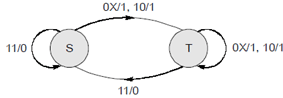The logic gate represented by the state diagram is

Solution: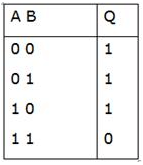If any one of the inputs is zero, output is logic ‘1’. Otherwise output is logic ‘0’, which represents the NAND gate.

QUESTION: 52

Consider the set of integers A defined as: {x∣1 ≤ x ≤ 123,n not divisible by 2,3 or 5} What is the cardinality of the power set of this set?

Solution:

Let X: items divisible by 2,Y: divisible by 3,z: divisible by 5 So, total number of elements in the set

X + Y + Z − (X∩Y) − (X∩Z) − (Y∩Z) + (X∩Y∩Z) Calculate this, it would come out to be 33 . Now, the power set is just 2n where n is the number of elements here 33. Hence, the answer 233

QUESTION: 53

There are five buildings A, B, C, D, E in a row. You are given the following statements:

E is to the east of C and west of A A is to the west of B

B is to the west of D

Which building is in the middle?

Solution:

As E is east of C and westof A: Hence, C E A A is to the west of B: C E A B

B is the west of D: C E A B D

QUESTION: 54

A relation on the integers 0 through 4 is defined by:

R = {(x, y): x + y ≤ 2x}

Which of the properties listed below applies to this relation?

I. Transitivity

II. Symmetry

III. Reflexivity

Solution:

Relation R on integer {0, 1, 2, 3, 4} is defined as

R = {(x, y) x + y ≤ 2x}

Reflexive relations : Reflexive relation on the set is a binary element in which every element is related to itself.

Transitive relation: Let A be a set in which the relation R defined. R is said to be transitive, if

(a, b) ∈ R and (b, a) ∈ R ⇒ (a, c) ∈ R,

That is aRb and bRc ⇒ aRc where a, b, c ∈ A

symmetric relation: Let A be a set in which the relation R defined. Then R is said to be a symmetric relation, if (a, b) ∈ R ⇒ (b, a) ∈ R, that is, aRb ⇒ bRa for all (a, b) ∈ R.

So, possible set of elements are

R = { (0, 0), (1, 0), (1, 1), (2, 0), (2, 1), (2, 2), (3, 0), (3, 1), (3, 2), (3, 3), (4, 0), (4, 1), (4, 3), (4, 4)}

From the set it is clear that relation is reflexive and transitive but it is not symmetric.

QUESTION: 55

In the set {1, 2, 3} a relation R = {(1, 2), (2, 3)}. How many more members must be included in R so that R will be an equivalence relation?

Solution:

Any relation is said to be equivalence if the relation is reflexive, symmetric and transitive.

The minimum number of elements that must be added is 7. 3 for reflexive property, 2 for symmetric {(2, 1), (3, 2)}. As (1,2) R (2,3); (1, 3) must be added for transitivity. Then (3,1) will also have to be included. Total 7 additions hence.

QUESTION: 56

The following system of homogeneous equations

2x + y + 2z = 0

x + y + 3z = 0

4x + 3y + bz = 0

has a non-trivial solution, then the value of ‘b’ is __.

Solution: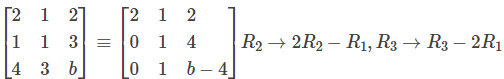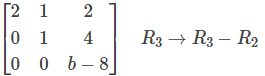If solution to be non trivial then rank of matrix should be less than number of variables

If b − 8= 0 than solution will be non trivial

So, B = 8

QUESTION: 57

The problem 3-SAT and 2-SAT are

Solution:

The Boolean satisfiability problem (SAT) is a decision problem, whose instance is a Boolean expression written using only AND, OR, NOT, variables, and parentheses. The problem is: given the expression, is there some assignment of TRUE and FALSE values to the variables that will make the entire expression true? A formula of propositional logic is said to be satisfiable if logical values can be assigned to its variables in a way that makes the formula true.

3-SAT and 2-SAT are special cases of k-satisfiability (k-SAT) or simply satisfiability (SAT), when each clause contains exactly k = 3 and k = 2 literals respectively.

2-SAT is P while 3-SAT is NP Complete.

QUESTION: 58

A semaphore S is an integer variable that, apart from initialization, is accessed only through two standard atomic operations: wait () and signal (). Thus which of the following in regards to semaphore is false.

Solution:

Semaphore variable requires busy waiting, While a process is in its critical section, any other process that tries to enter its critical section must loop continuously in the entry code.

SOLUTION TO THE BUSY WAITING PROBLEM

To overcome the need for busy waiting, we can modify the definition of the wait () and signal () semaphore operations. When a process executes the wait () operation and finds that the semaphore value is not positive, it must wait. However, rather than engaging in busy waiting, the process can block itself. The block operation places a process into a waiting queue associated with the semaphore, and the state of the process is switched to the waiting state. Then control is transferred to the CPU scheduler, which selects another process to execute. A process that is blocked, waiting on a semaphore S, should be restarted when some other process executes a signal ( ) operation. The process is restarted by a wakeup () operation, which changes the process from the waiting state to the ready state.

QUESTION: 59

If a system has 3 frames and following references are given in demand paging. Find the number of page faults using FIFO. Assume initially all three frames are empty.

Reference order:e, d, h, b, d, e, d, a, e, b, e, d, e, b, g.

Solution:

11 page faults using FIFO.

QUESTION: 60

The virtual and Physical addresses are same and different in respectively which address-binding schemes

Solution:

QUESTION: 61

Consider a virtual system with 2 level paging 32 bit virtual address is used to support 32 bit physical address, Page size is 4096 byte pages and page table has 4 bytes of page table entry. How many memory accesses are required to read or write a single word.

Solution: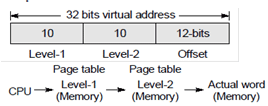3 memory accesses are required.

QUESTION: 62

Consider the following PDA transmissions

1: δ(q0,q,z0) Ã (q0,Xz0)

2: δ(q0,a,X) Ã (q0,X)

3: δ(q0,b,X) Ã (q1,∈)

4: δ(q1,b,z0) Ã (q1,z0)

5: δ(q1, ∈,z0) Ã (q1, ∈)

Where Q={q0,q1}, ∑ = {a,b} , Γ = {z , X} , δ, q0 , z0 , F= {φ}

What is the language accepted by the above push down machine?

Solution:

It will push ‘X’ for first ‘a’ and all other a’s will be bypassed. Whenever b encounters it will pop X and bypass all other b’s.

QUESTION: 63

Match the following groups.

List-I (Finite Automata)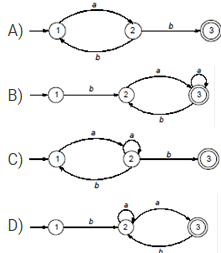List-II (Regular Expression)

1. b(a+ab)*a

2. a(a+ba)*b

3. ba(a+ba)*

4. a(ba)*b

Solution:

A-4, B-3, C-2, D-1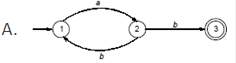Equivalent regular expressions are : I(ab)*ab and II a(ba)*b ..(4)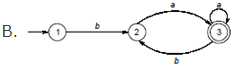Equivalent regular expression are: I b(aa*b)*aa* and II ba(a+ba)* …(3)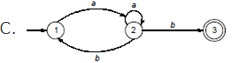Equivalent regular expressions are: I a(a+ba)*b and II (aa*b)*aa*b ..(2)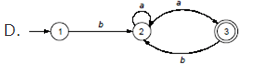Equivalent regular expressions are: I b(a+ab)*a and II ba*a(ba*a)* …(1)

QUESTION: 64

A tree with vertices is called graceful, if its vertices can be labelled with integers 1, 2, …..such that the absolute value of the difference of the labels of adjacent vertices are all different. Which of the following trees are graceful?

Solution:

Caterpillar tree: In graph theory, a caterpillar is a tree in which all the vertices are within distance 1 of a central path. Theorem: All caterpillars are graceful.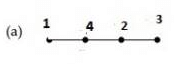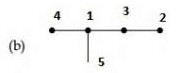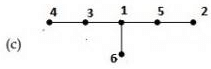QUESTION: 65

Let a × H and b × H be two cosets of H.

i. Either a × H and b ∗ H are disjoint

ii. a × H and b × H are identical

Then

Solution:

Both the left and right cosets are either completely disjoint or they can be completely identical to one another.

Hence only option C satisfies the condition as other than this the possibilities are none.Use Code STAYHOME200 and get INR 200 additional OFF Use Coupon Code